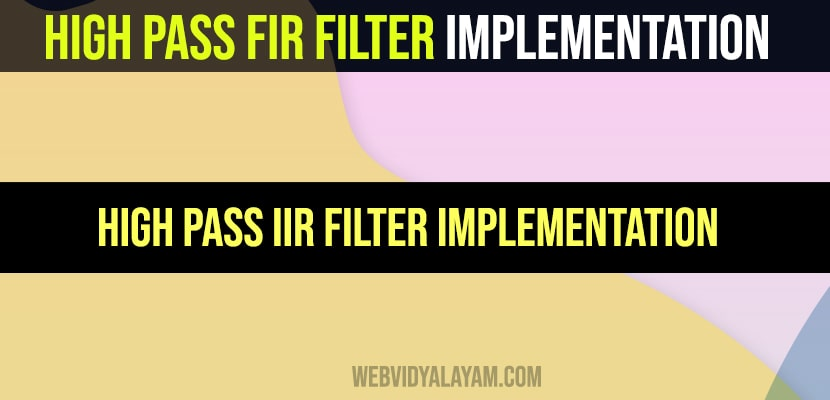# High pass FIR filter implementation and High pass IIR filter implementation

Steps involved in performing FIR filter operation

Step I: Let’s enter the pass band frequency (fp) and stop band frequency (fq).

Step II: And then get the sampling frequency (fs), length of window (n).

Step III: Next calculate cut off frequency.

Step IV: Use the boxcar, hamming, Blackman Commands for designing window.

Step V: And then design filter by using above parameters.

Step VI: To find frequency response of the filter by using matlab command freqz.

Step VII: Then plot the magnitude response and phase response of the filter.

Program:

clc;

clear all;

close all;

n=20;

fp=300;

fq=200;

fs=1000;

fn=2*fp/fs;

window=blackman(n+1);

b=fir1(n,fn,’high’,window);

[H W]=freqz(b,1,128);

subplot(2,1,1);

plot(W/pi,abs(H));

title(‘mag res of lpf’);

ylabel(‘gain in db——–>’);

xlabel(‘normalized frequency——>’);

subplot(2,1,2);

plot(W/pi,angle(H));

title(‘phase res of lpf’);

ylabel(‘angle——–>’);

xlabel(‘normalized frequency——>’);

Steps involved in performing IIR filter operation

Step I: Now enter the pass band ripple (rp) and stop band ripple (rs).

Step II: while the pass band frequency (wp) and stop band frequency (ws).

Step III: Then Get the sampling frequency (fs).

Step IV: Then calculate the normalized pass band frequency, and normalized stop band frequency w1 and w2 respectively.

Step V : Make using the following function for calculating the Butterworth filter order [n,wn]=buttord(w1,w2,rp,rs ) and Chebyshev filter order [n,wn]=cheb1ord(w1,w2,rp,rs)

Step VI: Let’s design the nth order digital high pass Chebyshev or Butterworth filter using the following commands. Butterworth filter [b,a]=butter (n, wn,’high’) Chebyshev filter [b,a]=cheby1 (n, 0.5, wn,’high’)

Step VII: In order to find the digital frequency response of the filter by using ‘freqz ( )’ function

Step VIII: Then calculate the magnitude for the frequency response in decibels (dB) mag=20*log10 (abs (H))

Step IX: Then plot the magnitude response [magnitude in dB Vs normalized frequency]

Step X: Next calculate the phase response using angle (H).

Step XI: Then plot the phase response [phase in radians Vs normalized frequency (Hz)].

Program:

clc;

clear all;

close all;

disp(‘enter the IIR filter design specifications’);

rp=input(‘enter the passband ripple’);

rs=input(‘enter the stopband ripple’);

wp=input(‘enter the passband freq’);

ws=input(‘enter the stopband freq’);

fs=input(‘enter the sampling freq’);

w1=2*wp/fs;w2=2*ws/fs;

[n,wn]=buttord(w1,w2,rp,rs,’s’);

disp(‘Frequency response of IIR HPF is:’);

[b,a]=butter(n,wn,’high’,’s’);

w=0:.01:pi;

[h,om]=freqs(b,a,w);

m=20*log10(abs(h));

an=angle(h);

figure, subplot(2,1,1);plot(om/pi,m);

title(‘magnitude response of IIR filter is:’);

xlabel(‘(a) Normalized freq. –>’);

ylabel(‘Gain in dB–>’);

subplot(2,1,2);plot(om/pi,an);

title(‘phase response of IIR filter is:’);

xlabel(‘(b) Normalized freq. –>’);